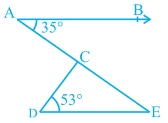Q

# In Fig. 6.41, if AB || DE, ∠ BAC = 35° and ∠ CDE = 53°, find ∠ DCE.

3.   In Fig. 6.41, if AB  DE, BAC = 35° and CDE = 53°, find DCE.ViewsWe have,
AB || DE,  BAC = 35° and  CDE = 53°

AE is a transversal so,  BAC =  AED =

Now, In  CDE,
CDE + DEC + ECD =  (By angle sum property)
Therefore, ECD =

Exams
Articles
Questions